# Exam-Style Questions on Integration

## Problems on Integration adapted from questions set in previous Mathematics exams.

### 1.

IB Standard

The graph of $$f(x)=8-x^2$$ crosses the x-axis at the points A and B.

(a) Find the x-coordinate of A and of B.

(b) The region enclosed by the graph of $$f$$ and the x-axis is revolved 360o about the x-axis. Find the volume of the solid formed.

### 2.

IB Standard

The acceleration, $$a$$ ms-2 , of an object at time $$t$$ seconds is given by

$$a=\frac1t+4sin3t, (t\ge1)$$

The object is at rest when $$t=1$$.

Find the velocity of the object when $$t=7$$.

### 3.

IB Analysis and Approaches

Find:

$$\int^{16}_9 \frac{6-2\sqrt{x}}{\sqrt{x}} dx$$

by first writing the algebraic fraction in the form $$ax^b+c$$.

### 4.

IB Analysis and Approaches

(a) Find $$\int (4x+5) dx$$.

(b) Given $$f'(x) = 4x+5$$ find $$f(x)$$ if $$f(3.4) = 10.12$$.

### 5.

IB Analysis and Approaches

Given that $$\frac{dy}{dx} = \sin(x + \frac{\pi}{3})$$ and $$y = 5$$ when $$x = \frac{8\pi}{3}$$, find $$y$$ in terms of $$x$$.

### 6.

IB Analysis and Approaches

The diagram below shows part of the graph of $$y = \dfrac{2x}{9-x^2}$$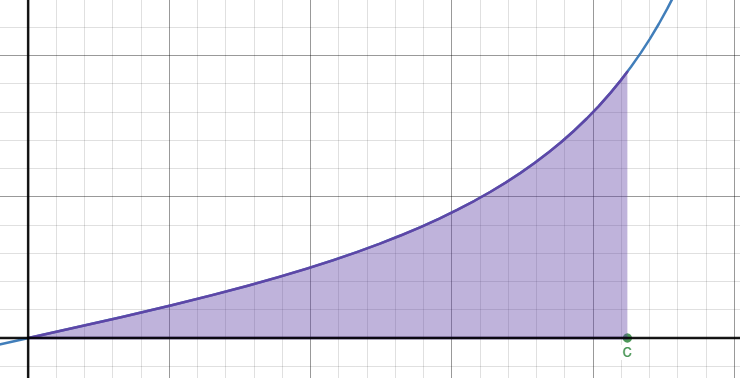The shaded region is bounded by the curve, the x-axis and the line $$x = c$$

The area of this region is $$\ln{2}$$

Find the value of $$c$$.

### 7.

IB Analysis and Approaches

The function $$f$$ is defined by $$f(x) = 8 - 5 \sin{x}$$, for $$x \ge 0$$.

The diagram shows part of the graph of $$y = f (x)$$.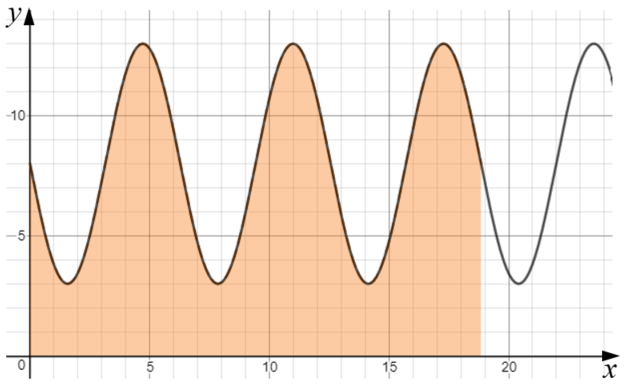The shaded region is enclosed by the graph of $$y=f(x)$$ and the x-axis for the first three periods of the function.

(a) Find the exact value of the x-coordinate of the right side of the shaded region.

(b) Show that the area of the shaded region is $$48 \pi$$.

A hemisphere has a total surface area in square centimetres equal to the shaded area in the previous diagram.

(c) Find the radius of the hemisphere.

### 8.

IB Applications and Interpretation

The following diagram shows part of the graph of:

$$f (x) = \frac{(9 + 4x) (6 - x)}{10}, x \in \mathbf R$$

The shaded region A is bounded by the x-axis, y-axis and the graph of $$f$$.(a) Write down an integral for the area of region A.

(b) Find the area of region A.

The three points A (0 , 0) , B (6 , 9) and C (p , 0) define the vertices of a triangle.

(c) Find the value of p, the x-coordinate of C , such that the area of the triangle is equal to the area of region A.

### 9.

IB Standard

Consider the graph of the function $$f(x)=x^2+2$$.

(a) Find the area between the graph of $$f$$ and the x-axis for $$2\le x \le 3$$.

(b) If the area described above is rotated 360o around the x-axis find the volume of the solid formed.

### 10.

A-Level

(a) Express the algebraic fraction

$$\frac{6x^2 - 47x + 49}{(5-x)(1-2x)}$$

in the form

$$A + \frac{B}{5-x} + \frac{C}{1-2x}$$

where $$A$$, $$B$$ and $$C$$ are integers.

(b) Hence show that the following integral equates to 3.03 correct to three significant figures.

$$\int^{0.25}_0 \frac{6x^2 - 47x + 49}{(5-x)(1-2x)} dx$$

### 11.

IB Standard

Make a sketch of a graph showing the velocity (in $$ms^{-1}$$) against time of a particle travelling for six seconds according to the equation:

$$v=e^{\sin t}-1$$

(a) Find the point at which the graph crosses the $$t$$ axis.

(b) How far does the particle travel during these first six seconds?

### 12.

IB Standard

Find the value of $$a$$ if $$\pi \lt a \lt 2\pi$$ and:

$$\int_\pi^a sin3x dx = -\frac13$$

### 13.

IB StandardThis graph represents the function $$f:x\to a \cos x, a\in \mathbf N$$

(a) Find the value of $$a$$.

(b) Find the area of the shaded region.

### 14.

IB Standard

Find $$f(x)$$ if $$f'(x)=6 \sin2x$$ and the graph of $$f(x)$$ passes through the point $$(\frac{\pi}{3},11)$$.

### 15.

IB Analysis and Approaches

The curve $$y=\sin(\sqrt{x}) \text{ where } x \ge 0$$ intersects the x axis at the points $$x_0, x_1, x_2, x_3, x_4, ...$$ and $$x_0 = 0$$.

(a) Find $$x_1$$, the first x-intercept of the function to the right of the origin. Give your answer in terms of $$\pi$$.

(b) Find an expression for the nth x-intercept of the curve, in terms of $$\pi$$.

(c) By using an appropriate substitution, show that:

$$\int \sin(\sqrt{x}) \; dx = 2\sin(\sqrt{x}) - 2 \sqrt{x} \cos(\sqrt{x})$$

The area of the region bounded by the curve and the x-axis is denoted by $$R_n$$ where:

$$R_n = \int^{x_{n+1}}_{x_n} y \; dx$$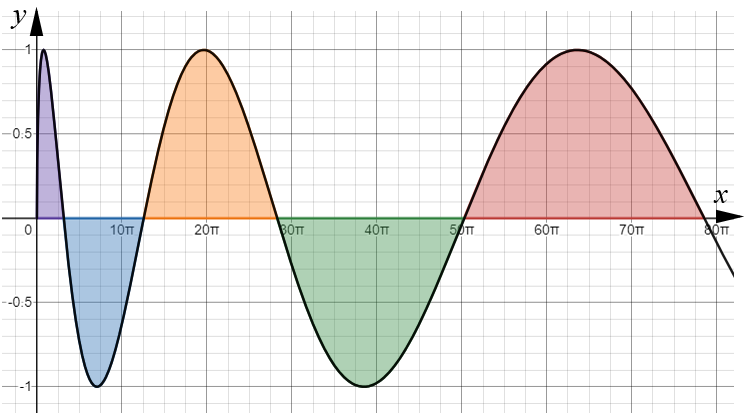(d) Calculate the area of region $$R_n$$ giving your answer in terms of $$\pi$$.

(e) Hence, show that the areas of the regions bounded by the curve and the x-axis, form an arithmetic sequence.

### 16.

A-Level

The diagram shows a sketch of the curve C with equation: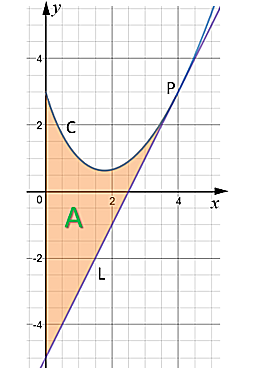$$y=2x^{\frac32}-4x+3$$

The point P with coordinates (4, 3) lies on C.

The line L is the tangent to C at the point P.

The region A, shown shaded, is bounded by the curve C, the line L and the y-axis.

Find the area of A making your method clear.

Note that solutions based entirely on graphical or numerical methods are not acceptable.)

### 17.

IB Standard

The following diagram shows the graph of $$f(x) = \cos(e^x) \; \text{for} \; 0 \le x \le 0.5$$.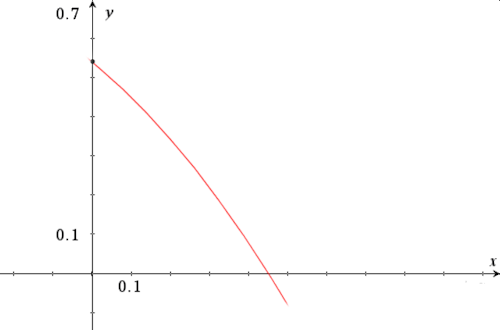(a) Find the x-intercept of the graph of $$f(x)$$.

The region enclosed by the graph of $$f(x)$$, the y-axis and the x-axis is rotated 360° about the x-axis.

(b) Find the volume of the solid formed.

### 18.

IB Analysis and Approaches

Find $$f(x)$$ if:

$$f'(x) = \frac{12x}{\sqrt{3x^2+4}}$$

given that $$f(0) = 10$$

If you would like space on the right of the question to write out the solution try this Thinning Feature. It will collapse the text into the left half of your screen but large diagrams will remain unchanged.

The exam-style questions appearing on this site are based on those set in previous examinations (or sample assessment papers for future examinations) by the major examination boards. The wording, diagrams and figures used in these questions have been changed from the originals so that students can have fresh, relevant problem solving practice even if they have previously worked through the related exam paper.

The solutions to the questions on this website are only available to those who have a Transum Subscription.

Exam-Style Questions Main Page

Search for exam-style questions containing a particular word or phrase:

To search the entire Transum website use the search box in the grey area below.